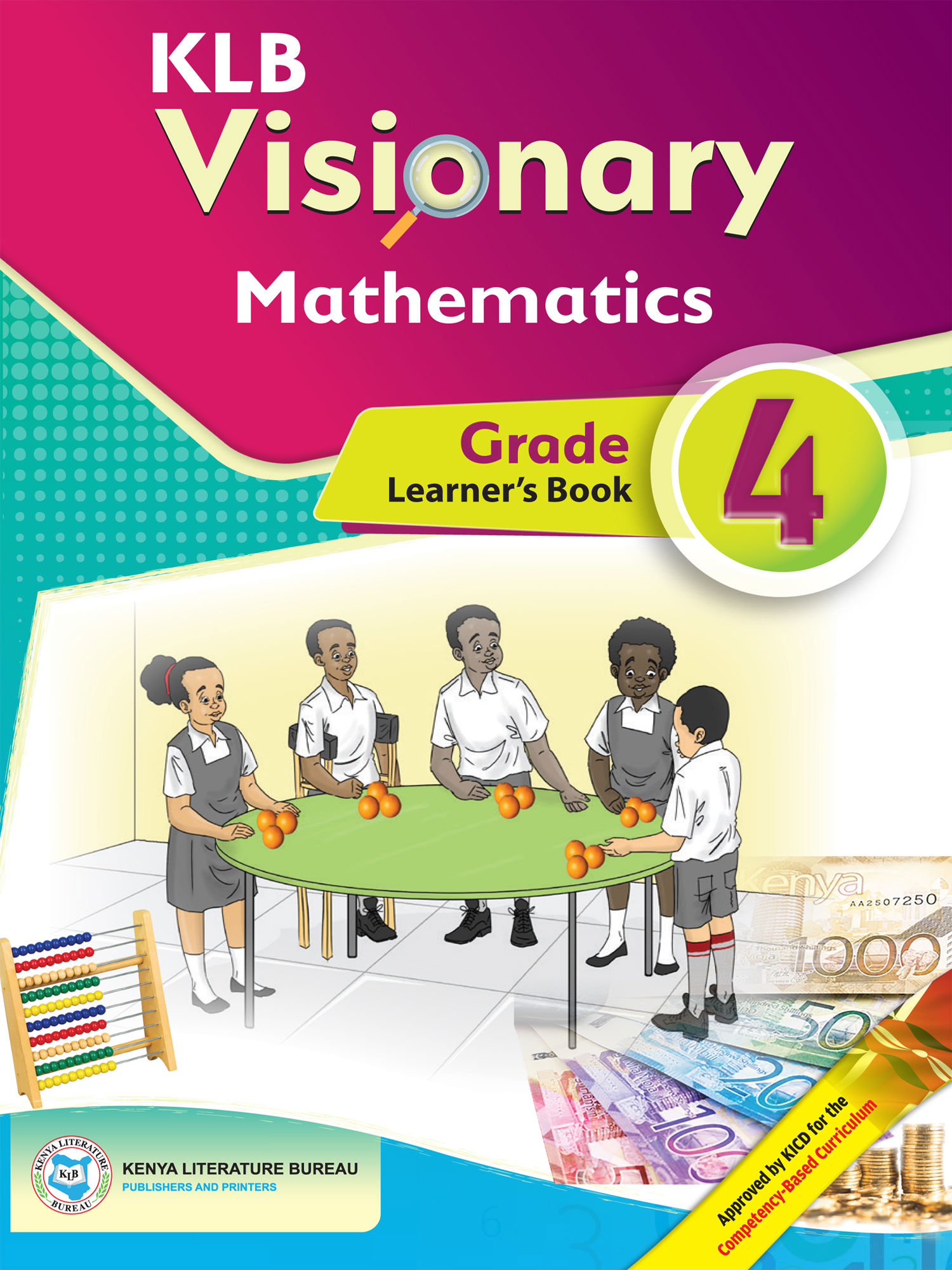Study kit (Mathematics 4th)##### Authors
Obadiah Kiburi, Samuel Irumbi, Zipporah N. Muli, Francis Mwangi
##### Exercises kit’s authors
Obadiah Kiburi, Samuel Irumbi, Zipporah N. Muli, Francis Mwangi
##### Publisher
Kenya Literature Bureau
##### Included in packages• The study kit contains 113 chapters and teacher’s exercises kit contains 195 exercises.
• ##### Authors
Obadiah Kiburi, Samuel Irumbi, Zipporah N. Muli, Francis Mwangi
• ##### Exercises kit’s authors
Obadiah Kiburi, Samuel Irumbi, Zipporah N. Muli, Francis Mwangi
Mathematics
English
• ##### Publisher
Kenya Literature Bureau

### 1. Numbers – Whole numbers

##### Chapter
1.1. Place value of numbers up to tens of thousands
Free chapter!
1.2. Identifying total values of digits up to tens of thousands
1.3. Reading and writing numbers up to 10 000 in symbols
1.4. Reading and writing numbers up to 1 000 in words
1.5. Ordering numbers up to 1 000
1.6. Rounding off numbers up to 500 to the nearest 10
1.7. Rounding off numbers up to 1 000 to the nearest 10
1.8. Factors and divisors of numbers up to 50
1.9. Multiples of numbers up to 100
1.10. Even and odd numbers up to 100
1.11. Patterns involving even and odd numbers
1.12. Roman numerals

##### Chapter
2.1. Addition of a 4-digit number up to a 2-digit number with single regrouping
2.2. Addition of a 4-digit number to a 3-digit and 4-digit number with single regrouping
2.3. Addition of a 4-digit number up to 3-digit with double regrouping
2.4. Addition of two 4-digit numbers with double regrouping
2.5. Finding sum by rounding off numbers to the nearest 10

### 3. Numbers – Subtraction

##### Chapter
3.1. Subtraction of up to 3-digit numbers without regrouping
3.2. Subtraction of up to 4-digit number without regrouping
3.3. Subtraction of up to 3-digit numbers with regrouping
3.4. Subtraction of up to 4-digit numbers with regrouping
3.5. Finding differences by rounding off numbers to the nearest tens
3.6. Number patterns involving subtraction

### 4. Numbers – Multiplication

##### Chapter
4.1. Multiplication of up to 2-digit numbers by multiples of 10
4.2. Multiplication of 1-digit numbers by multiples of 10
4.3. Multiplication of 2-digit number by 1-digit number without regrouping
4.4. Multiplication of 2-digit number by 1-digit number with regrouping
4.5. Multiplication of a 2-digit number by multiples of 10
4.6. Multiplication of a 2-digit number by a 2-digit number without regrouping
4.7. Multiplication of a 2-digit number by a 2-digit number with regrouping
4.8. Estimating products by rounding off numbers to the nearest 10
4.9. Number patterns involving multiplication

### 5. Numbers – Division

##### Chapter
5.1. Division of 2-digit number by 1-digit number without remainder
5.2. Division of up to 2-digit number by 1-digit number with remainder
5.3. The long form of division
5.4. Division using different methods
5.5. Relationship between multiplication and division

### 6. Numbers – Fractions

##### Chapter
6.1. Showing and writing fractions: part of a whole
6.2. Showing and writing fractions: part of a group
6.3. Numerator and denominator
6.4. Types of fractions
6.5. Converting improper fractions to mixed fractions and vice versa

### 7. Numbers – Decimals

##### Chapter
7.1. Writing tenths and hundredths
7.2. Writing tenths using decimal notation
7.3. Writing hundredths using decimal notation
7.4. Place value of decimals: tenths
7.5. Place value of decimals: hundredths
7.6. Ordering tenths
7.7. Ordering hundredths

### 8. Measurement – Length

##### Chapter
8.1. Measuring length
8.2. Measuring lines
8.3. Estimate and actual length
8.4. Converting units of length
8.5. Perimeter
8.6. Addition of units of length
8.7. Subtraction of units of length
8.8. Multiplication of units of length
8.9. Division of units of length

### 9. Measurement – Area

##### Chapter
9.1. Area of surfaces
9.2. Area of squares and rectangles by counting unit squares
9.3. Finding area by multiplying rows and columns
9.4. Area of square and rectangle

### 10. Measurement – Mass

##### Chapter
10.1. Measuring mass using 1 kg and ½ kg masses
10.2. Measuring mass using ¼ kg masses
10.3. Mass in ½ kg and ¼ kg
10.5. Subtraction of mass

### 11. Measurement – Volume

##### Chapter
11.1. Cube and cuboid
11.2. Cube
11.3. Cuboid

### 12. Measurement – Capacity

##### Chapter
12.1. Capacity in litres and ½ litres
12.2. Capacity in ¼ litres
12.3. Capacity in ½ litres and ¼ litres
12.5. Subtraction of capacity

### 13. Measurement – Time

##### Chapter
13.1. Time in a.m. and p.m.
13.2. Estimating time
13.3. Converting hours to minutes and vice versa
13.4. Converting days to hours and vice versa
13.5. Converting weeks to days and vice versa
13.6. Measuring time during events
13.7. Addition of units of time
13.8. Subtraction of units of time

### 14. Measurement – Money

##### Chapter
14.1. Conversion of units of money
14.2. Change
14.3. Balance
14.4. Needs and wants
14.5. Savings
14.7. Subtraction of money
14.8. County Government money

### 15. Geometry – Position and Direction

##### Chapter
15.1. Clockwise and anti‑clockwise turns
15.2. A quarter, half and full turn

### 16. Geometry – Angles

##### Chapter
16.1. An angle
16.2. Types of angles
16.3. Comparing angles

### 17. Geometry – Shapes

##### Chapter
17.1. Shapes in our environment
17.2. Lines of symmetry
17.3. Properties of square and rectangle
17.4. Properties of triangles
17.5. Making patterns

### 18. Data Handling

##### Chapter
18.1. Collecting and organising data
18.2. Tally marks
18.3. Showing data
18.4. Interpretation (1)
18.5. Interpretation (2)